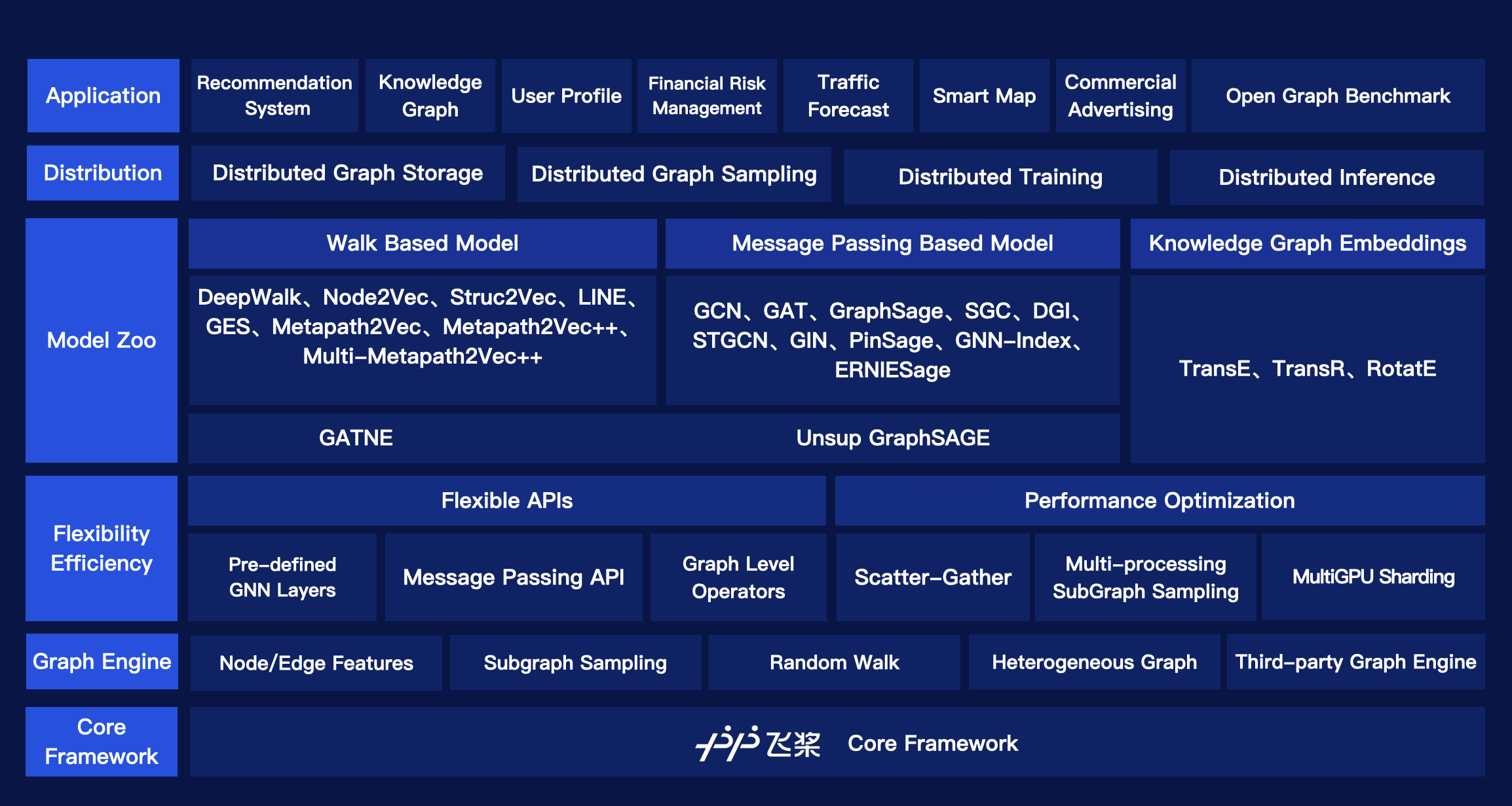The Framework of Paddle Graph Learning (PGL)

The newly released PGL supports heterogeneous graph learning on both walk based paradigm and message-passing based paradigm by providing MetaPath sampling and Message Passing mechanism on heterogeneous graph. Furthermor, The newly released PGL also support distributed graph storage and some distributed training algorithms, such as distributed deep walk and distributed graphsage. Combined with the PaddlePaddle deep learning framework, we are able to support both graph representation learning models and graph neural networks, and thus our framework has a wide range of graph-based applications.

One of the most important benefits of graph neural networks compared to other models is the ability to use node-to-node connectivity information, but coding the communication between nodes is very cumbersome. At PGL we adopt Message Passing Paradigm similar to DGL to help to build a customize graph neural network easily. Users only need to write send and recv functions to easily implement a simple GCN. As shown in the following figure, for the first step the send function is defined on the edges of the graph, and the user can customize the send function $$\phi^e$$ to send the message from the source to the target node. For the second step, the recv function $$\phi^v$$ is responsible for aggregating $$\oplus$$ messages together from different sources.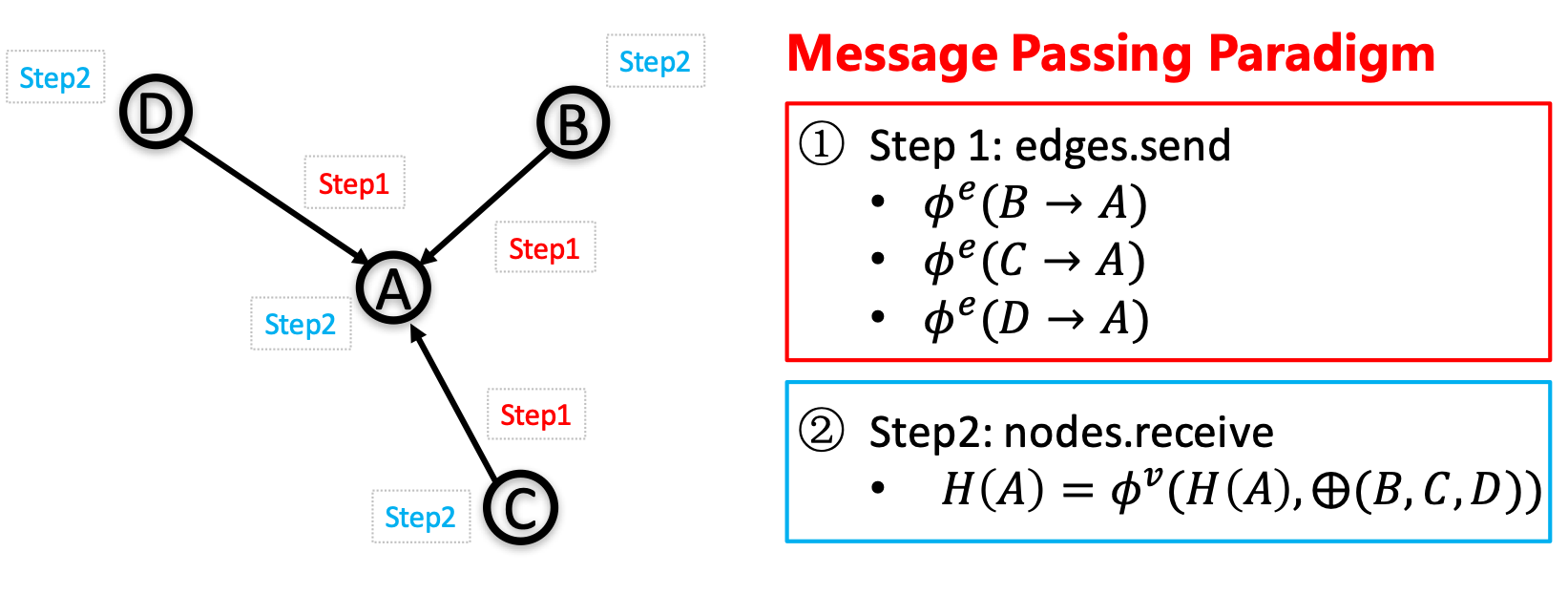The basic idea of message passing paradigm

To write a sum aggregator, users only need to write the following codes.

import pgl
import numpy as np

num_nodes = 5
edges = [(0, 1), (1, 2), (3, 4)]
feature = np.random.randn(5, 100).astype(np.float32)

g = pgl.Graph(num_nodes=num_nodes,
edges=edges,
node_feat={
"h": feature
})
g.tensor()

def send_func(src_feat, dst_feat, edge_feat):
return src_feat

def recv_func(msg):
return msg.reduce_sum(msg["h"])

msg = g.send(send_func, src_feat=g.node_feat)

ret = g.recv(recv_func, msg)


## Highlight: Flexibility - Natively Support Heterogeneous Graph Learning¶

Graph can conveniently represent the relation between things in the real world, but the categories of things and the relation between things are various. Therefore, in the heterogeneous graph, we need to distinguish the node types and edge types in the graph network. PGL models heterogeneous graphs that contain multiple node types and multiple edge types, and can describe complex connections between different types.

### Support meta path walk sampling on heterogeneous graph¶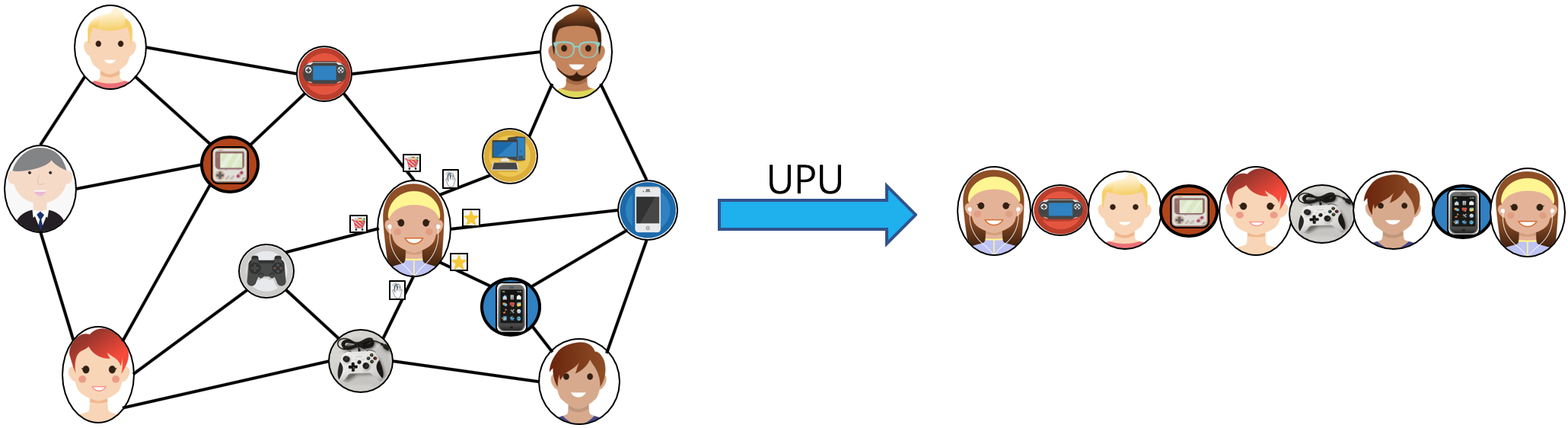The metapath sampling in heterogeneous graph

The left side of the figure above describes a shopping social network. The nodes above have two categories of users and goods, and the relations between users and users, users and goods, and goods and goods. The right of the above figure is a simple sampling process of MetaPath. When you input any MetaPath as UPU (user-product-user), you will find the following results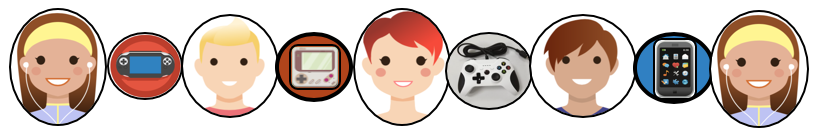The metapath result

Then on this basis, and introducing word2vec and other methods to support learning metapath2vec and other algorithms of heterogeneous graph representation.

### Support Message Passing mechanism on heterogeneous graph¶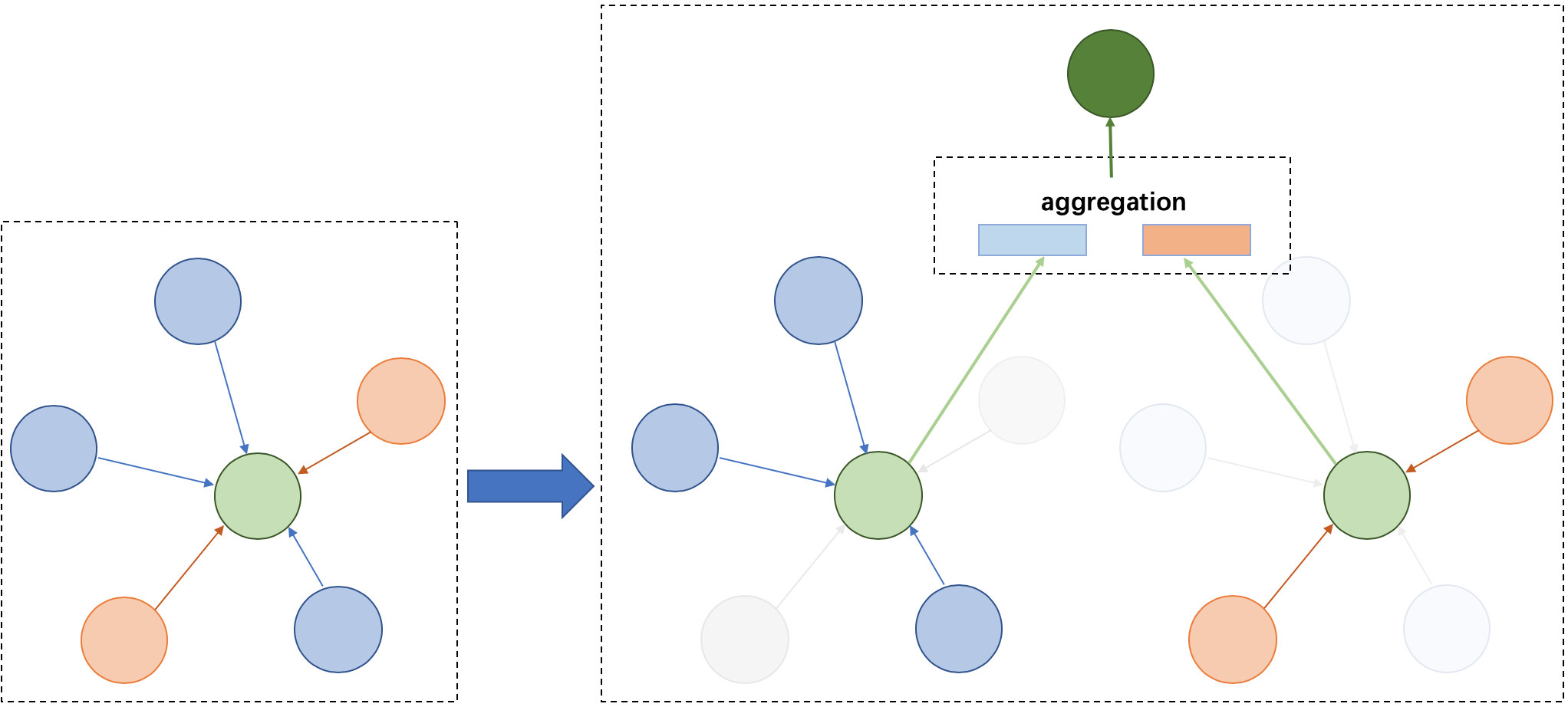The message passing mechanism on heterogeneous graph

Because of the different node types on the heterogeneous graph, the message delivery is also different. As shown on the left, it has five neighbors, belonging to two different node types. As shown on the right of the figure above, nodes belonging to different types need to be aggregated separately during message delivery, and then merged into the final message to update the target node. On this basis, PGL supports heterogeneous graph algorithms based on message passing, such as GATNE and other algorithms.

## Large-Scale: Support distributed graph storage and distributed training algorithms¶

In most cases of large-scale graph learning, we need distributed graph storage and distributed training support. As shown in the following figure, PGL provided a general solution of large-scale training, we adopted PaddleFleet as our distributed parameter servers, which supports large scale distributed embeddings and a lightweighted distributed storage engine so tcan easily set up a large scale distributed training algorithm with MPI clusters.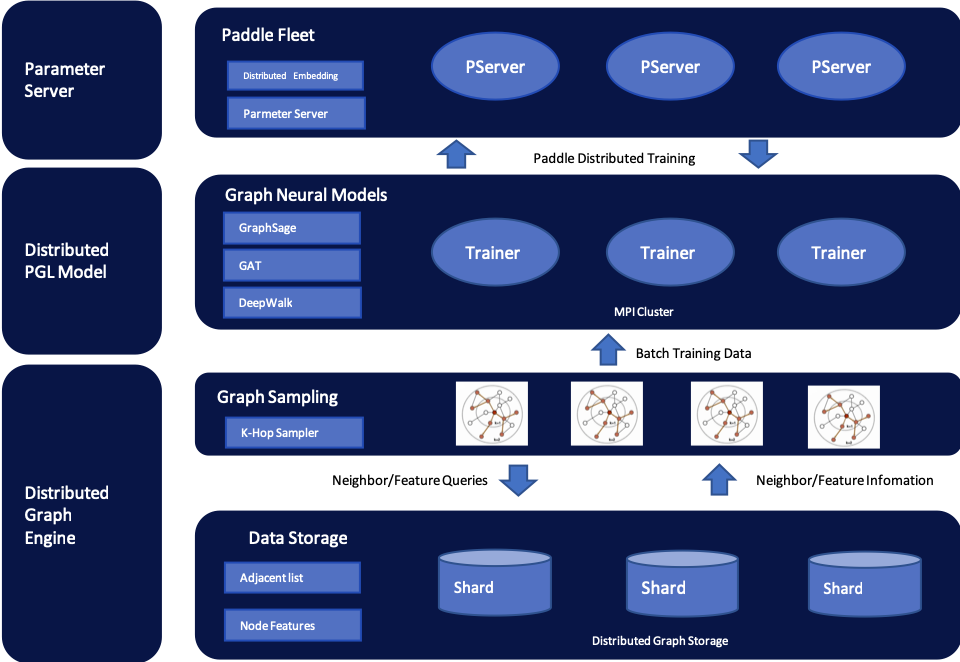The distributed frame of PGL

## Model Zoo¶

The following graph learning models have been implemented in the framework. You can find more examples and the details here.

Model

feature

ERNIESage

ERNIE SAmple aggreGatE for Text and Graph

GCN

Graph Convolutional Neural Networks

GAT

Graph Attention Network

GraphSage

Large-scale graph convolution network based on neighborhood sampling

unSup-GraphSage

Unsupervised GraphSAGE

LINE

Representation learning based on first-order and second-order neighbors

DeepWalk

Representation learning by DFS random walk

MetaPath2Vec

Representation learning based on metapath

Node2Vec

The representation learning Combined with DFS and BFS

Struct2Vec

Representation learning based on structural similarity

SGC

Simplified graph convolution neural network

GES

The graph represents learning method with node features

DGI

Unsupervised representation learning based on graph convolution network

GATNE

Representation Learning of Heterogeneous Graph based on MessagePassing

The above models consists of three parts, namely, graph representation learning, graph neural network and heterogeneous graph learning, which are also divided into graph representation learning and graph neural network.

## System requirements¶

PGL requires:

• cython

PGL only supports Python 3

## Installation¶

You can simply install it via pip.

pip install pgl


## The Team¶

PGL is developed and maintained by NLP and Paddle Teams at Baidu

E-mail: nlp-gnn[at]baidu.com